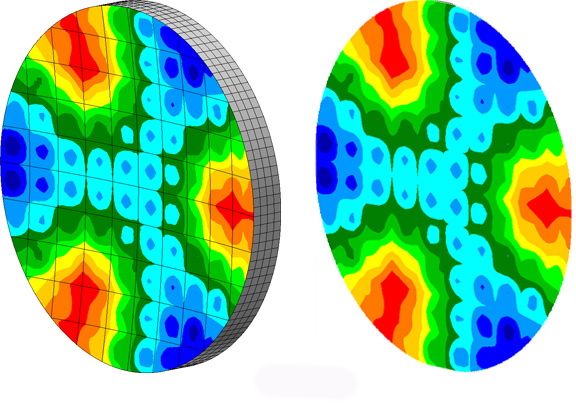### The interpolation feature in SigFit is used for (1) importing disturbance or actuator influence data from rectangular array data as might be obtained in an optical test, and (2) exporting SigFit analysis results data into a rectangular array format.

The capability to import data from optical test allows users to combine finite element analysis results with test data. For example, a user can simulate the adaptive correction of an imperfectly figured optic obtained by importing into SigFit adaptive control feature optical test data as a disturbance and finite element simulations of influence functions as actuators. Any results may be formatted into user defined rectangular array formats so that they may be subsequently compared to test data in other test correlation software.Figure 1 – Deformation dominated by quilting requires interpolation instead of polynomial fitting for surface deformation characterization. The surface deformation in the figure on the left is accurately represented by the array data shown on the right.

Interpolation in SigFit uses finite element shape functions to ensure that the finite element description of the deformation is most accurately charcterized. The user may choose to use linear or quadratic shape functions depending on the elements used to construct the finite element model